Home
Hostname: page-component-8bbf57454-q5g9d Total loading time: 0.231 Render date: 2022-01-24T09:54:21.929Z Has data issue: true Feature Flags: { "shouldUseShareProductTool": true, "shouldUseHypothesis": true, "isUnsiloEnabled": true, "metricsAbstractViews": false, "figures": true, "newCiteModal": false, "newCitedByModal": true, "newEcommerce": true, "newUsageEvents": true }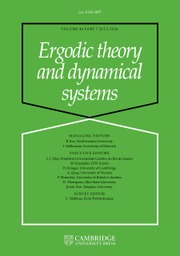Ergodic Theory and Dynamical Systems

Area-preserving diffeomorphisms of the torus whose rotation sets have non-empty interior

Published online by Cambridge University Press:  13 August 2013

Abstract

In this paper we consider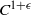${C}^{1+ \epsilon }$ area-preserving diffeomorphisms of the torus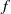$f$, either homotopic to the identity or to Dehn twists. We suppose that$f$ has a lift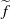$\widetilde {f}$ to the plane such that its rotation set has interior and prove, among other things, that if zero is an interior point of the rotation set, then there exists a hyperbolic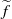$\widetilde {f}$-periodic point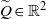$\widetilde {Q} \in { \mathbb{R} }^{2}$ such that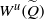${W}^{u} (\widetilde {Q} )$ intersects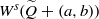${W}^{s} (\widetilde {Q} + (a, b))$ for all integers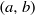$(a, b)$, which implies that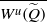$\overline{{W}^{u} (\widetilde {Q} )}$ is invariant under integer translations. Moreover,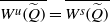$\overline{{W}^{u} (\widetilde {Q} )} = \overline{{W}^{s} (\widetilde {Q} )}$ and$\widetilde {f}$ restricted to$\overline{{W}^{u} (\widetilde {Q} )}$ is invariant and topologically mixing. Each connected component of the complement of$\overline{{W}^{u} (\widetilde {Q} )}$ is a disk with diameter uniformly bounded from above. If$f$ is transitive, then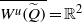$\overline{{W}^{u} (\widetilde {Q} )} = { \mathbb{R} }^{2}$ and$\widetilde {f}$ is topologically mixing in the whole plane.

Type
Research Article
Information
Ergodic Theory and Dynamical Systems , February 2015 , pp. 1 - 33

Access options

Get access to the full version of this content by using one of the access options below. (Log in options will check for institutional or personal access. Content may require purchase if you do not have access.)

References

Addas-Zanata, S.. On the existence of a new type of periodic and quasi-periodic orbits for twist maps of the torus. Nonlinearity 15 (2002), 13991416.CrossRefGoogle Scholar
Addas-Zanata, S.. A note on a standard family of twist maps. Qual. Theory Dyn. Syst. 5 (2004), 19.CrossRefGoogle Scholar
Addas-Zanata, S.. Some extensions of the Poincaré–Birkhoff theorem to the cylinder and a remark on mappings of the torus homotopic to Dehn twists. Nonlinearity 18 (2005), 22432260.CrossRefGoogle Scholar
Addas-Zanata, S. and Tal, F.. Homeomorphisms of the annulus with a transitive lift. Math. Z. 267 (2011), 971980.CrossRefGoogle Scholar
Addas-Zanata, S., Garcia, B. and Tal, F.. On the dynamics of homeomorphisms of the torus homotopic to Dehn twists. Ergod. Th. & Dynam. Sys., to appear. doi: 10.1017/etds.2012.156.CrossRefGoogle Scholar
Boyland, P.. Isotopy stability of dynamics on surfaces. Contemp. Math. 246 (1999), 1745.CrossRefGoogle Scholar
Brown, M.. Homeomorphisms of two-manifolds. Houston J. Math. 11 (1985), 455469.Google Scholar
de Carvalho, A. and Paternain, M.. Monotone quotients of surface diffeomorphisms. Math. Res. Lett. 10 (2003), 603619.CrossRefGoogle Scholar
Doeff, E.. Rotation measures for homeomorphisms of the torus homotopic to a Dehn twist. Ergod. Th. & Dynam. Sys. 17 (1997), 117.CrossRefGoogle Scholar
Duarte, P.. Plenty of elliptic islands for the standard family of area preserving maps. Ann. Inst. H. Poincaré Anal. Non Linéaire 11 (1994), 359409.CrossRefGoogle Scholar
Epstein, D.. Curves on 2-manifolds and isotopies. Acta Math. 115 (1966), 83107.CrossRefGoogle Scholar
Fathi, A., Laudenbach, F. and Poenaru, V.. Travaux de Thurston sur les surfaces. Astérisque. 66–67 (1979).Google Scholar
Franks, J.. Realizing rotation vectors for torus homeomorphisms. Trans. Amer. Math. Soc. 311 (1989), 107115.CrossRefGoogle Scholar
Franks, J. and Le Calvez, P.. Regions of instability for non-twist maps. Ergod. Th. & Dynam. Sys. 23 (2003), 111141.CrossRefGoogle Scholar
Guelman, N., Koropecki, A. and Tal, F.. (2013), Rotation sets with non-empty interior and transitivity in the universal covering. Ergod. Th. & Dynam. Sys., to appear.Google Scholar
Handel, M.. Global shadowing of pseudo-Anosov homeomorphisms. Ergod. Th. & Dynam. Sys. 5 (1985), 373377.CrossRefGoogle Scholar
Handel, M.. The rotation set of a homeomorphism of the annulus is closed. Comm. Math. Phys. 127 (1990), 339349.CrossRefGoogle Scholar
Handel, M. and Thurston, W.. New proofs of some results of Nielsen. Adv. Math. 56 (1985), 173191.CrossRefGoogle Scholar
Katok, A.. Lyapunov exponents, entropy and periodic orbits for diffeomorphisms. Publ. Math. Inst. Hautes Études Sci. 51 (1980), 137173.CrossRefGoogle Scholar
Katok, A. and Hasselblatt, B.. Introduction to the Modern Theory of Dynamical Systems. Cambridge University Press, Cambridge, 1995.CrossRefGoogle Scholar
Khinchin, A.. Continued Fractions. Dover edition, New York, 1997.Google Scholar
Koropecki, A. and Tal, F.. Strictly toral dynamics. Invent. Math., to appear.Google Scholar
Kwapisz, J.. Every convex polygon with rational vertices is a rotation set. Ergod. Th. & Dynam. Sys. 12 (1992), 333339.CrossRefGoogle Scholar
Kwapisz, J.. A toral diffeomorphism with a nonpolygonal rotation set. Nonlinearity 8 (1995), 461476.CrossRefGoogle Scholar
Kwapisz, J.. Rotation sets and entropy. PhD Thesis, 1995, SUNY at Stony Brook, USA.Google Scholar
Kwapisz, J. and Swanson, R.. Asymptotic entropy, periodic orbits, and pseudo-Anosov maps. Ergod. Th. & Dynam. Sys. 18 (1998), 425439.CrossRefGoogle Scholar
Llibre, J. and Mackay, R.. Rotation vectors and entropy for homeomorphisms of the torus isotopic to the identity. Ergod. Th. & Dynam. Sys. 11 (1991), 115128.CrossRefGoogle Scholar
Misiurewicz, M. and Ziemian, K.. Rotation sets for maps of tori. J. Lond. Math. Soc. 40 (1989), 490506.CrossRefGoogle Scholar
Thurston, W.. On the geometry and dynamics of diffeomorphisms of surfaces. Bull. Amer. Math. Soc. 19 (1988), 417431.CrossRefGoogle Scholar
Walters, P.. An Introduction to Ergodic Theory (Graduate Texts in Mathematics, 79). Springer, New York–Berlin, 1982.CrossRefGoogle Scholar
6
Cited by

Send article to Kindle

Note you can select to send to either the @free.kindle.com or @kindle.com variations. ‘@free.kindle.com’ emails are free but can only be sent to your device when it is connected to wi-fi. ‘@kindle.com’ emails can be delivered even when you are not connected to wi-fi, but note that service fees apply.

Find out more about the Kindle Personal Document Service.

Area-preserving diffeomorphisms of the torus whose rotation sets have non-empty interior
Available formats
×

Send article to Dropbox

To send this article to your Dropbox account, please select one or more formats and confirm that you agree to abide by our usage policies. If this is the first time you use this feature, you will be asked to authorise Cambridge Core to connect with your <service> account. Find out more about sending content to Dropbox.

Area-preserving diffeomorphisms of the torus whose rotation sets have non-empty interior
Available formats
×

To send this article to your Google Drive account, please select one or more formats and confirm that you agree to abide by our usage policies. If this is the first time you use this feature, you will be asked to authorise Cambridge Core to connect with your <service> account. Find out more about sending content to Google Drive.

Area-preserving diffeomorphisms of the torus whose rotation sets have non-empty interior
Available formats
×
×